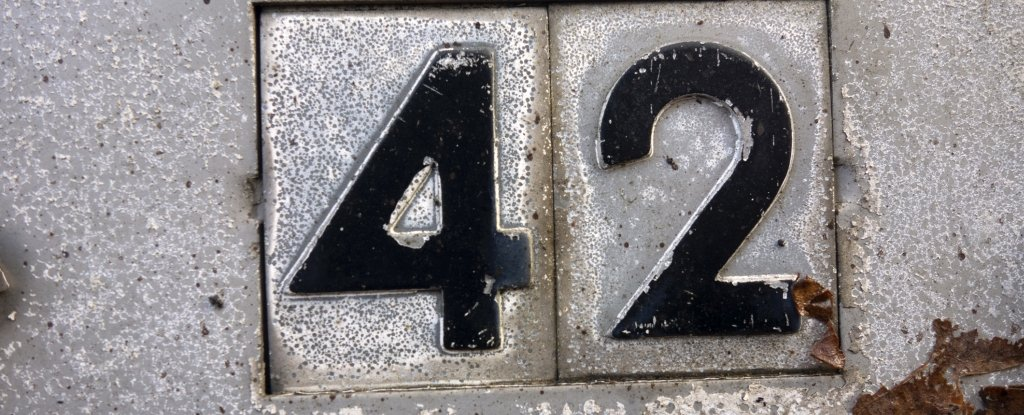(Michelle Still Artist/iStock)

# Mathematicians Solve '42' Problem With Planetary Supercomputer

MICHELLE STARR
9 SEPTEMBER 2019

Mathematicians have finally figured out the three cubed numbers that add up to 42. This has settled a problem that has been pondered for 65 years: namely, can each of the natural numbers below 100 be expressed as the sum of three cubes?

The problem, set in 1954, is exactly what it sounds like: x3+y3+z3=k. K is each of the numbers from 1 to 100; the question is, what are x, y and z?

Over the following decades, solutions were found for the easier numbers. In 2000, mathematician Noam Elkies of Harvard University published an algorithm to help find the harder ones.

This year, just the two most difficult ones remained: 33 and 42.

Then, after watching a YouTube video about the problem with 33 on the popular maths channel Numberphile, mathematician Andrew Booker from the University of Bristol in the UK was inspired to write a new algorithm. He ran this through a powerful supercomputer at the university's Advanced Computing Research Centre, and got the solution for 33 after just three weeks.

So, we were left with the hardest one of them all: 42. This proved a much more obstinate problem, so Booker enlisted the aid of fellow MIT mathematician Andrew Sutherland, an expert in massively parallel computation.

As you already know from the headline of this article, they figured it out. They also did a fun reveal of their success: according to The Aperiodical, both mathematicians quietly changed their personal websites to the solution, and named the pages "Life, the Universe, and Everything", a fitting nod to Douglas Adams.

Of course, it wasn't simple. The pair had to go large, so they enlisted the aid of the Charity Engine, an initiative that spans the globe, harnessing unused computing power from over 500,000 home PCs to act as a sort of "planetary supercomputer".

It took over a million hours of computing time, but the two mathematicians found their solution.

X = -80538738812075974

Y = 80435758145817515

Z = 12602123297335631

So, the full equation is (-80538738812075974)3 + 804357581458175153 + 126021232973356313 = 42.

"I feel relieved," Booker said.

"In this game, it's impossible to be sure that you'll find something. It's a bit like trying to predict earthquakes, in that we have only rough probabilities to go by. So, we might find what we're looking for with a few months of searching, or it might be that the solution isn't found for another century."

Is that it, then? Well… no. That's just 1 to 100 covered. Go up an order of magnitude to 1,000, and there are still plenty of numbers to solve - 114, 165, 390, 579, 627, 633, 732, 906, 921 and 975 are all awaiting a solution to the sum of three cubes.

Got any ideas?